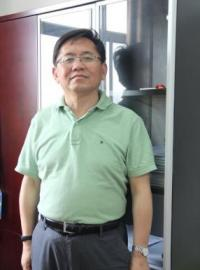Analyticity and Inverse Problems for PDE
Speaker
Prof. Jin Cheng
School of Mathematical Sciences, Fudan University
Abstract

Analyticity is a fundamental property in Calculus and Complex Analysis. It is closely related with the properties of the solutions of differential equations. But this property is rarely used in the scientific computing. In this talk, I will show that the analyticity of the solutions for partial differential equations can be used to study the inverse and ill-posed problems for PDE. It is a powerful tool for solving the incomplete data problems. Several examples are presented.